## Search Here

### FORMULAS FOR FIND AREA

Square =  a2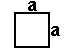• Rectangle =  ab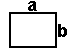• Parallelogram =  bh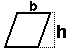• Trapezoid =  h/2 (b1 + b2)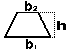• Circle =  π r 2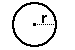• Ellipse = π r1 r2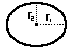• Triangle = (1/2) b h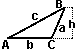• Equilateral triangle =  (1/4)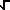(3) a2

• Triangle given SAS =  (1/2) a b sin C

Triangle given a,b,c[s(s-a)(s-b)(s-c)] when s = (a+b+c)/2(Heron's formula)

Regular polygon = (1/2) n sin(360°/n) S2
When n = Number of sides and S = length from center to a corner

<< Formulas For Finding Volume